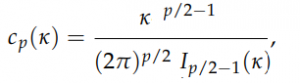# Von Mises Fisher Distribution

Share on

The Von Mises Fisher Distribution (vMF) is an important isotropic distribution for directional data and statistics. “Directional” statistics are simply statistics that have direction as well as magnitude, like gene expression data, wind current directions or measurements taken from compasses (Dhillon & Sra, 2003).

The von Mises Fisher Distribution is a generalization of the von Mises Distribution to higher dimensions. However, this very simple distribution bears many similarities to the multivariate normal distribution. This includes the fact that the vMF distribution, like the normal distribution, arises naturally in many situations. For example directional data falls naturally into a vMF distribution, as does data distributed on the unit hypersphere (Banerjee et al., 2005).

## Formal Definition for the Von Mises Fisher Distribution

A unit norm vector x has a von Mises-Fisher distribution when its density is:

Pvmf(x; μ κ) := cp(κ) e κμΤx

Where:

• Cp = the normalizing constant,
• ||μ|| = 1 and κ > 0. (Sra, 2016)
• x ∈ Sp-1 (also written as Sp, this is the p dimensional unit hypersphere).

The normalizing constant (found by integrating polar coordinates) is given by:Where Is(Κ) is the modified Bessel function of the first kind.

## References

Banerjee, A. et al. (2005). Clustering on the Unit Hypersphere using von Mises-Fisher Distributions. Journal of Machine Learning Research 6 (2005) 1345–1382.
Dhillon, I. & Sra, S. (2003). Modeling Data using Directional Distributions. Retrieved January 15, 2020 from: https://www.cs.utexas.edu/users/inderjit/public_papers/tr03-06.pdf
Sra, S. (2016). Directional Statistics in Machine Learning: a Brief Review. Retrieved January 15, 2019 from: http://arxiv-export-lb.library.cornell.edu/pdf/1605.00316

CITE THIS AS:
Stephanie Glen. "Von Mises Fisher Distribution" From StatisticsHowTo.com: Elementary Statistics for the rest of us! https://www.statisticshowto.com/von-mises-fisher-distribution/
---------------------------------------------------------------------------Need help with a homework or test question? With Chegg Study, you can get step-by-step solutions to your questions from an expert in the field. Your first 30 minutes with a Chegg tutor is free!